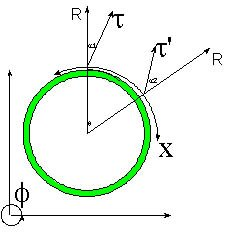# Hypergeometrical Universe

## Why is the Speed of Light the Speed Limit?Why is the Speed of Light the Speed Limit?

I asked myself this question many times. Einstein simply hypothesized that the speed of light is the same for all inertial reference frames. This hypothesis results in the speed of light being the speed limit in our 3-D Universe (4-D spacetime). This is not an explanation on the why is the speed of light the speed bump of our Universe, it just an observation of how things are.

It is certainly not a simple and clear explanation...:)

Look at the ubiquitous picture above...:)

The circle above is a shock wave where any direction in our Universe can be mapped to the perimeter X. The shock wave Universe is traveling at the obligatory speed of light along the direction R, which is a Radial direction perpendicular to our X direction and thus to our 3-D Universe.

The first thing to realize is that the speed of light is not the highest velocity one can travel, it is the ONLY velocity one can travel.

Traveling at the speed of light along our 3-D Universe is equivalent to riding the interference of two spacetime waves: One that is our 3-D Shock Wave Universe and another perpendicular to the Radial direction.

The resulting interference would land our traveler on a 45 degrees trajectory with respect to the Radial direction and traveling at the speed of light...:)

Simple and Clear...

Currently unrated

or